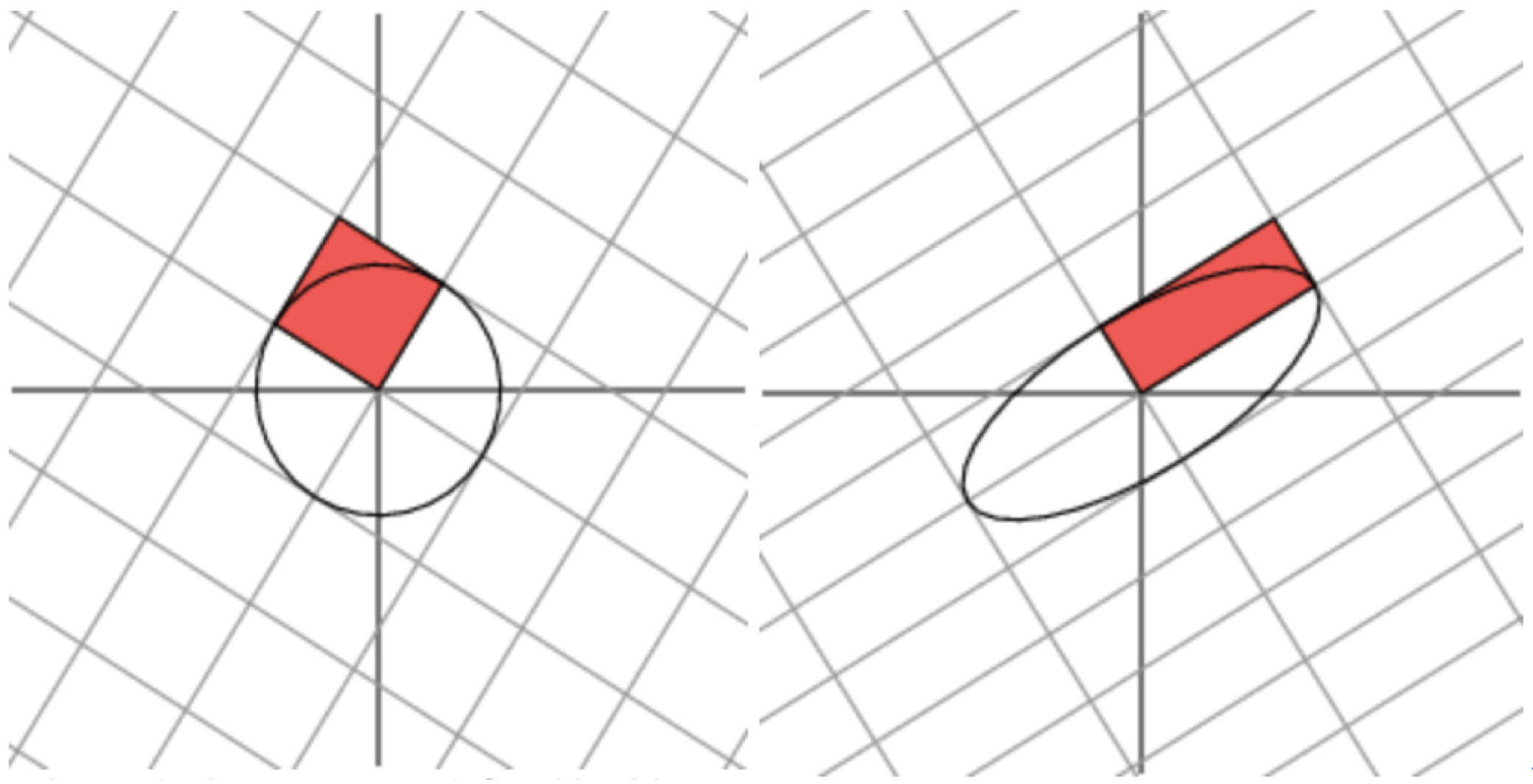# 一、特征值分解（EVD）

## 1.1 特征值分解、特征值、特征向量

Av=λv$Av = \lambda v$

(λIA)v=0$(\lambda I-A)v = 0$

det|λIA|=0$det|\lambda I-A |= 0$ (此式被称为矩阵A的特征多项式)我们可以得到下式：

(λλ1)n1(λλ2)n2(λλl)nl=0$(\lambda - \lambda _{1})^{n_{1}}(\lambda - \lambda _{2})^{n_{2}}\cdot \cdot \cdot (\lambda - \lambda _{l})^{n_{l}} = 0$

li=1ni=N$\sum_{i = 1}^{l}n_{i} = N$

A=QΛQ1$A = Q\Lambda Q^{-1}$

## 1.2 特征向量的求解

• 计算A的特征多项式det|λIA|=0$det|\lambda I-A |= 0$，从而求得特征值 λi$\lambda_{i}$

• 对于单根特征值来说，求齐次方程 (λiIA)vi=0$(\lambda_{i} I-A)v_{i} = 0$，即可求得该特征值对应的特征向量

• 对于有重根的特征值来说，可以使用一下公式，依次迭代求解

v1:Av1=λv1$v_{1} : Av_{1} = \lambda v_{1}$

v2:Av2=v1+λv2$v_{2} : Av_{2} =v_{1} + \lambda v_{2}$

v3:Av3=v2+λv3$v_{3} : Av_{3} =v_{2} + \lambda v_{3}$

$\cdot \cdot \cdot$

vN:AvN=vN1+λvN$v_{N} : Av_{N} =v_{N-1} + \lambda v_{N}$

## 1.3 特征值与特征向量的意义解释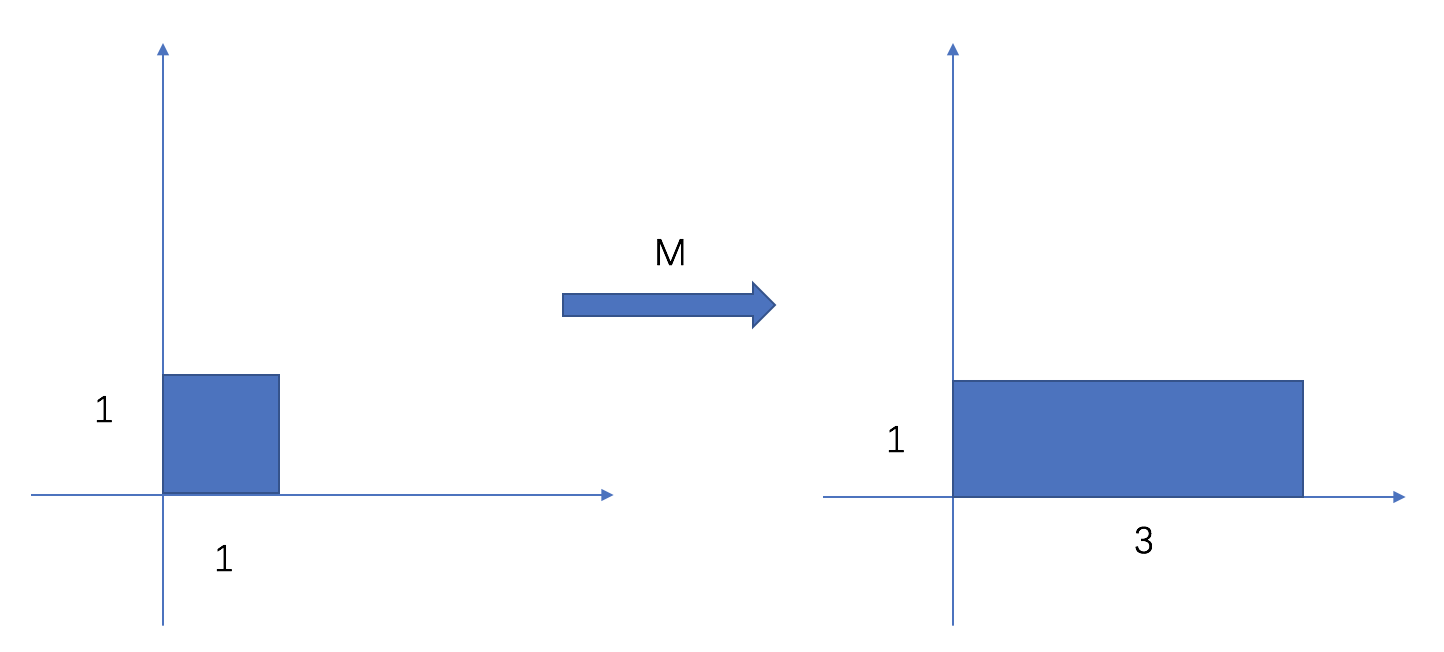M矩阵是对称的，所以这个变换是一个对x,y轴的拉伸变换，此处将原方块在x轴方向拉长了三倍。对于不是对称的情况，如 M=$M= \begin{bmatrix}1 & 1 \\ 0 & 1 \end{bmatrix}$ ，对应的线性变换为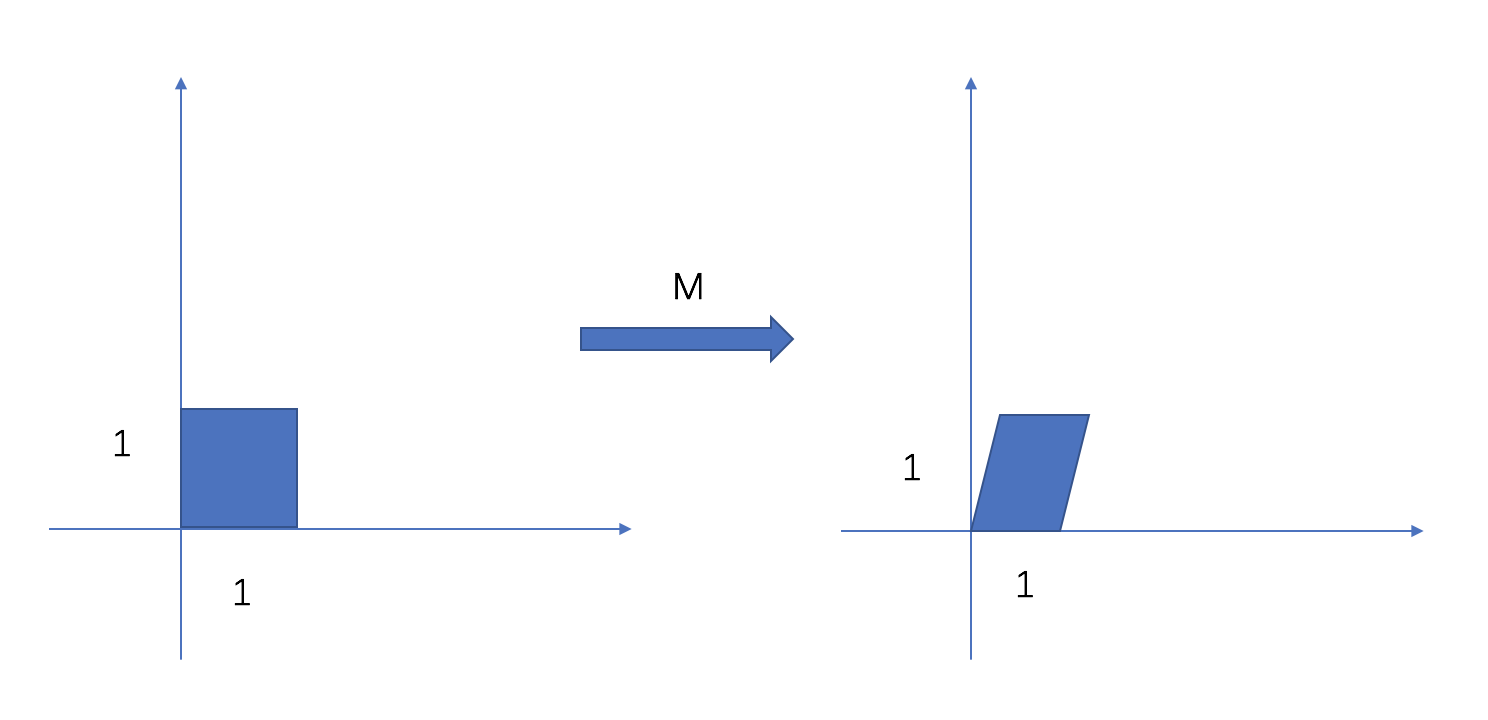# 二、相似对角化

## 2.1 相似矩阵的定义

• 自反性：A～A
• 对称性：若A～B，则B～A
• 传递性：若A～B，B～A，则A～C

## 2.2 相似对角化的条件与推论

N阶方阵A与对角阵相似的前提是：A有N个线性无关的特征向量。可以对角化即意味着存在某组基，使得这个矩阵所代表的线性变换在这组基的每一个方向上都是伸缩变换复向量上的复“伸缩变换“近似于在某种意义上非刚性但依然线性的伸缩旋转），不能对角化即意味着找不到这样一组基

• AΛ=diag(λ1,λ2,,λN)$A \sim \Lambda = diag(\lambda_{1},\lambda_{2},\cdot\cdot\cdot,\lambda_{N})$ ,则A与 Λ$\Lambda$ 的特征值相同，Λ$\Lambda$ 的主对角线元素 λ1,λ2,,λN$\lambda_{1},\lambda_{2},\cdot\cdot\cdot,\lambda_{N}$ 为A的全部特征值。相似变换的变换矩阵为 P=(p1,p2,,pN)$P = (p_{1},p_{2},\cdot\cdot\cdot,p_{N})$，则列向量p1,p2,,pN$p_{1},p_{2},\cdot\cdot\cdot,p_{N}$依次是λ1,λ2,,λN$\lambda_{1},\lambda_{2},\cdot\cdot\cdot,\lambda_{N}$对应的特征向量

• 相似变换矩阵不唯一，因为特征向量的排列顺序可以发生变化

• AΛ$A\sim\Lambda$，若不计Λi$\Lambda_{i}$的排列顺序，则Λ$\Lambda$唯一，称为A的相似标准型

### 2.2.2 推论二

λ1,λ2,,λN$\lambda_{1},\lambda_{2},\cdot\cdot\cdot,\lambda_{N}$是A的l$l$个互不相同的特征值，重数依次为r1,r2,,rN$r_{1},r_{2},\cdot\cdot\cdot,r_{N}$，且有r1+r2++rl=N$r_{1} + r_{2}+\cdot\cdot\cdot +r_{l} = N$,则A可以相似对角化的充分必要条件为：A的ri$r_{i}$重特征值λi$\lambda_{i}$恰有ri$r_{i}$个线性无关的特征向量(i=1,2,,l)$(i = 1,2,\cdot\cdot\cdot,l)$

### 2.2.3 推论三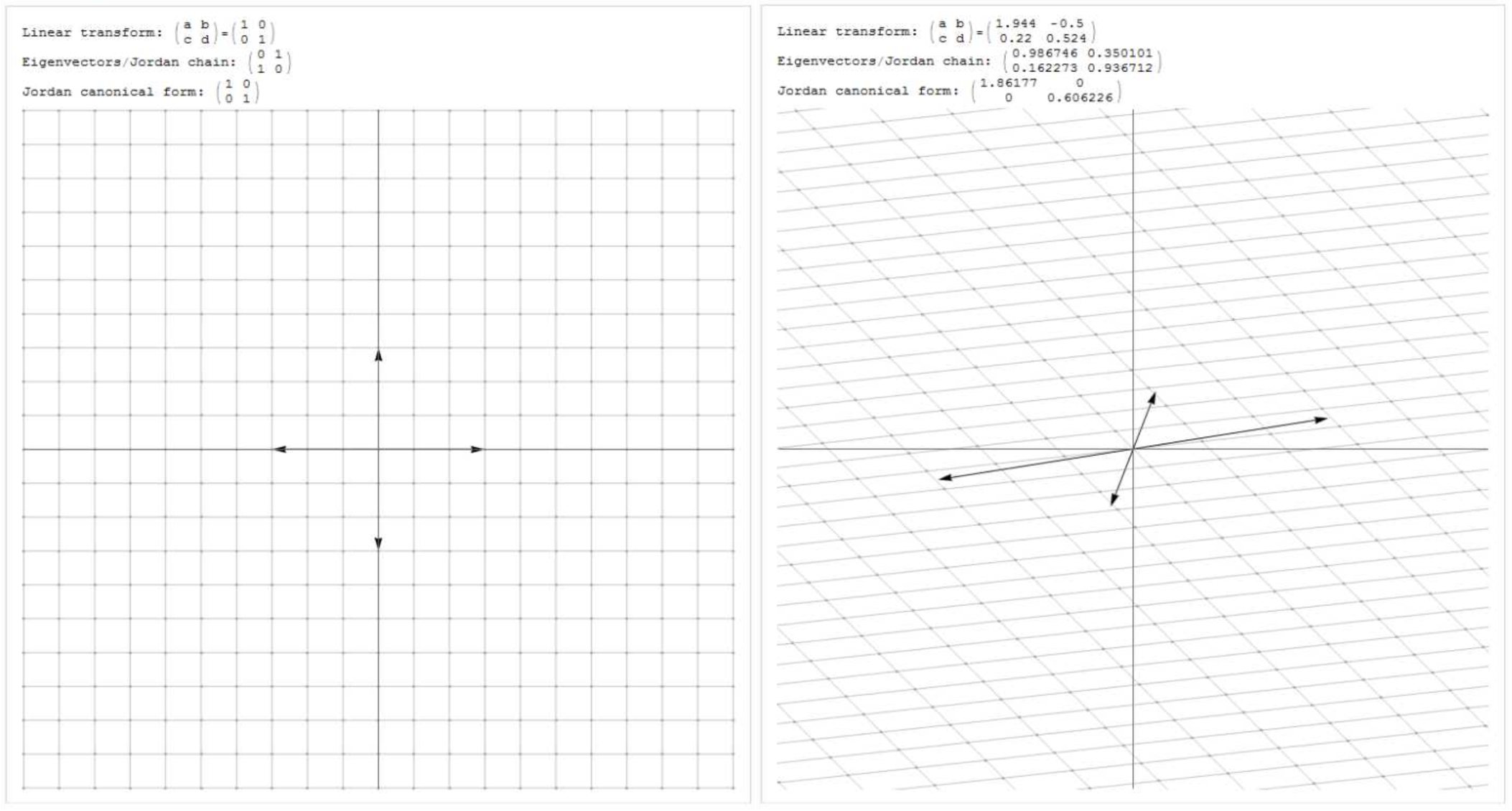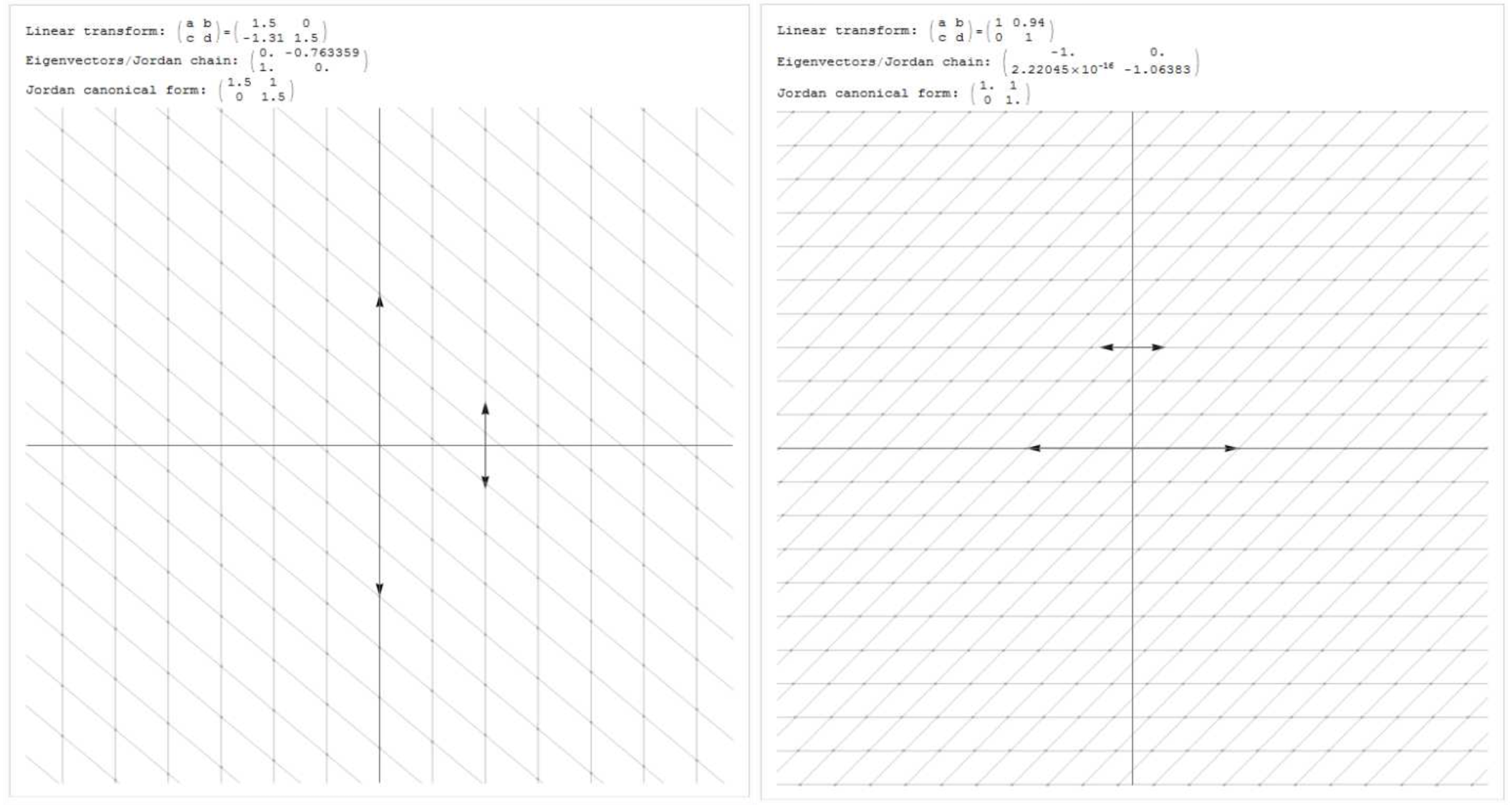## 2.3 实对称矩阵与相似对角化

### 2.3.1 实对称矩阵的特征值与特征向量

AT=A$A^{T} = A$ , A¯=A$\bar{A} = A$

Av=λv,v0$Av = \lambda v , v \neq 0$

v¯TAv=v¯TATv=(Av¯)Tv=(Av)Tv$\bar{v}^{T}Av = \bar{v}^{T}A^{T}v = (A\bar{v})^{T}v = (\overline{Av})^{T}v$

λv¯Tv=λ¯v¯Tv$\lambda \bar{v}^{T}v = \bar{\lambda} \bar{v}^{T}v$

λ=λ¯$\lambda = \bar{\lambda}$

λ$\lambda$为实数，因此det|AλE|x=0$det|A-\lambda E|x= 0$必有实的基础解系，从而对应的特征向量可以取实向量

### 2.3.2 实对称矩阵正交相似于对角矩阵

Q1AQ=QTAQ=Λ=diag(λ1,λ2,,λN)$Q^{-1}AQ = Q^{T}AQ = \Lambda = diag(\lambda_{1},\lambda_{2},\cdot\cdot\cdot,\lambda_{N})$

• 求出A的全部特征值
• 求出每个特征值所对应的全部特征向量
• 以特征向量为列向量写出变换矩阵
• 使用Schmidt 正交化将变换矩阵正交化，单位化，得到正交矩阵Q

## 2.4 相似对角化与特征值分解的区别

• 目的：特征值分解的目的在于矩阵分解，求得矩阵对应的特征值与特征向量；而相似对角化的目的在于通过变换矩阵的线性变换将原方阵转换为对角矩阵

• 条件：所有的方阵都可以进行特征值分解得到对应的特征值与特征向量；只有当方阵的几何重数与代数重数相等（方阵的最小多项式无重根）时，方阵才可以实现对角化

• 结果：通过特征值分解得到的特征向量与特征值可以构成对角标准型与jordan标准型（前者是后者的特例），其中Jordan标准型不是对角矩阵；而相似对角化得到的矩阵一定是对角矩阵

# 三、QR分解

QR分解是目前求取一般矩阵全部特征值的最有效并且广泛应用的办法,它是将矩阵分解成为一个正交矩阵Q和一个上三角矩阵R，所以称为QR分解。这一分解方法除了用于特征值计算外，在参数估计和通信领域也有着广泛的应用

## 3.1 QR分解的定义与推导

AϵRn×m$A \epsilon R^{n\times m}$，且 n>=m$n >= m$，则存在列正交矩阵 QϵRn×m$Q \epsilon R^{n\times m}$上三角矩阵 RϵRm×m$R \epsilon R^{m\times m}$，使得 A=QR$A=QR$
m=n$m=n$ 时 ，Q$Q$为正交矩阵。如果A是非奇异的n×n$n \times n$ 矩阵，则R的所有对角线元素均为正，并且在这种情况下，Q$Q$R$R$是唯一的。若A是复矩阵，则Q$Q$R$R$取复值

• A$A$ 表示为 A=[x1,x2,,xm]$A = [x_{1},x_{2},\cdot\cdot\cdot,x_{m}]$
• 由于A$A$满秩，所以 xi$x_{i}$ 之间线性独立，通过Schmidt 正交化我们可以得到一组正交向量和一个上三角矩阵如下

[u1u2um]=[x1x2xm]$[u_{1} u_{2}\cdot\cdot\cdot u_{m}] = [x_{1} x_{2}\cdot\cdot\cdot x_{m}]$t11000t1mtmm$\begin{bmatrix} t_{11} & \cdot\cdot\cdot & t_{1m} \\ 0 & \ddots & \vdots \\ 0 & 0 & t_{mm}\end{bmatrix}$

U=AT$U = AT$

• 这里的T矩阵是Schmidt 正交化的变换矩阵，由于
tii=xii1j=1<uj,xi>uj1$t_{ii} = \begin{Vmatrix} x_{i} - \sum_{j = 1}^{i -1}u_{j} \end{Vmatrix}^{-1}$

矩阵T是非奇异的，同时T1$T^{-1}$也同样为上三角矩阵，令Q=U$Q = U$R=T1$R = T^{-1}$,我们便可以得到A=QR$A = QR$

## 3.2 QR分解的应用

QR分解在实际工程问题中得到了广泛的应用，其核心还是围绕着利用QR分解求解矩阵的特征值进行的，这里列举出一些常见的例子

# 四、Schur分解

## 4.1 什么是酉矩阵？

### 4.1.1 “等距”(isometry)

<Ux,Uy>=<x,y>$ = $

Ux=<Ux,Uy>1/2=<x,y>1/2=x$\begin{Vmatrix} Ux \end{Vmatrix} = ^{1/2} = ^{1/2} = \begin{Vmatrix} x \end{Vmatrix}$

### 4.1.3 酉矩阵(unitary matrix)

UUH=UHU=I$UU^{H} = U^{H}U = I$

## 4.2 Schur分解的定义与推导

UHAU=T$U^{H}AU = T$

T$T$为一个上三角矩阵，它的对角线元素 tii=λi$t_{ii} = \lambda_{i}$。现在来证明Schur分解的存在

• xi$x_{i}$ 为对应于特征值 λi$\lambda_{i}$ 的特征向量，令 X1=[x1,x2,,xn]$X_{1} = [x_{1},x_{2},\cdot\cdot\cdot,x_{n}]$

• X1$X_{1}$ 进行QR分解，可以得到X1=Q1R1$X_{1} = Q_{1}R_{1}$Q1$Q_{1}$这里是酉矩阵，R1$R_{1}$是上三角矩阵。要注意的是 Q1$Q_{1}$ 的第一列仍然是 A$A$ 对应于特征值 λi$\lambda_{i}$ 的特征向量，因此有

QH1AQ1=[λ10A1]$Q_{1}^{H}AQ_{1} = \begin{bmatrix} \lambda_{1} & * \\ 0 & A_{1} \end{bmatrix}$

这里 A1ϵC(n1)×(n1)$A_{1} \epsilon C^{(n-1)\times(n-1)}$，它的特征值为 λ2,,λn$\lambda_{2},\cdot\cdot\cdot,\lambda_{n}$

• 使用同样的步骤，我们又可以得到一个酉矩阵 Q2ϵC(n1)×(n1)$Q_{2} \epsilon C^{(n-1) \times (n-1)}$，得到

QH2A1Q2=[λ20A2]$Q_{2}^{H}A_{1}Q_{2} = \begin{bmatrix}\lambda_{2} & * \\ 0 & A_{2} \end{bmatrix}$

再令
U2=[100Q2]$U_{2} = \begin{bmatrix} 1 & 0 \\ 0 & Q_{2} \end{bmatrix}$

于是有
U_{2}^{H}Q_{1}^{H}AQ_{1}U_{2} =
λ100λ20A2

-重复上述步骤，得到酉矩阵 QiϵC(ni+1)×(ni+1)$Q_{i} \epsilon C^{(n-i+1) \times (n-i+1)}$ 可以使

QHiAi1Qi=[λi0Ai]$Q_{i}^{H}A_{i-1}Q_{i} = \begin{bmatrix}\lambda_{i} & * \\ 0 & A_{i} \end{bmatrix}$

以及 UiϵCn×n$U_{i} \epsilon C^{n \times n}$
Ui=[I00Qi]$U_{i} = \begin{bmatrix} I & 0 \\ 0 & Q_{i} \end{bmatrix}$

• 最后矩阵U=Q1U2Un1$U = Q_{1}U_{2}\cdot\cdot\cdot U_{n-1}$即为所求的酉矩阵

## 4.3 Schur分解的缺陷

Schur分解将原方阵转化为了一个对角线为特征值的上三角矩阵，在这一章节的开头已经说过Schur分解是很多重要定理推导的基石与出发点。但是矩阵的Schur分解，在更多意义上是一种理论上的存在，在实际中通常不方便通过有限次运算得到，真正要计算时，一般也是通过迭代的方法进行逼近

# 五、奇异值分解（SVD）

## 5.1奇异值分解的定义与推导

A=UΛVT$A= U\Lambda V^{T}$

λ1>=λ2>=>=λmin(n,m)$\lambda_{1} >= \lambda_{2} >= \cdot\cdot\cdot >= \lambda_{min(n,m)}$

• 首先，我们对n阶对称方阵 ATA$A^{T}A$ 作特征值分解，得到

ATA=VΛVT$A^{T}A= V\Lambda V^{T}$

• 通过特征值分解我们得到一组正交基 V=(v1,v2,,vn)$V = (v_{1},v_{2},\cdot\cdot\cdot,v_{n})$，满足如下性质

(ATA)vi=λivi$(A^{T}A)v_{i} = \lambda_{i}v_{i}$

由于ATA$A^{T}A$为对称矩阵，vi$v_{i}$之间两两相互正交，所以有
<Avi,Avj>=vTi(ATA)vj=vTiλjvj=λjvTivj=0$ = v^{T}_{i}(A^{T}A)v_{j} = v^{T}_{i}\lambda_{j}v_{j} = \lambda_{j}v^{T}_{i}v_{j} = 0$

• 因为rank(ATA)=rank(A)=r$rank(A^{T}A) = rank(A) = r$，我们可以得到另一组正交基 Av1,Av1,,Avr${Av_{1},Av_{1},\cdot\cdot\cdot,Av_{r}}$将其标准化有

ui=Avi|Avi|=1λAvi$u_{i} = \frac{Av_{i}}{|Av_{i}|} = \frac{1}{\sqrt{\lambda}}Av_{i}$

Avi=λiui=δiui$Av_{i} = \sqrt{\lambda_{i}}u_{i} =\delta_{i}u_{i}$

注：
|Avi|2=<Avi,Avi>=λivTivi=λi$|Av_{i}|^{2}==\lambda_{i}v^{T}_{i}v_{i} = \lambda_{i}$

• 将向量组 (u1,u2,,ur)$(u_{1},u_{2},\cdot\cdot\cdot,u_{r})$扩充为Fm$F^{m}$中的标准正交基(u1,u2,,ur,,um)$(u_{1},u_{2},\cdot\cdot\cdot,u_{r},\cdot\cdot\cdot,u_{m})$则：

AV=A(v1,v2,,vn)=(Av1,Av2,,Avr,0,,0)=(δ1u1,δ2u2,,δrur,0,,0)=UΛ$AV = A(v_{1},v_{2},\cdot\cdot\cdot, v_{n}) = (Av_{1},Av_{2},\cdot\cdot\cdot,Av_{r},0,\cdot\cdot\cdot,0) = (\delta_{1}u_{1},\delta_{2}u_{2},\cdot\cdot\cdot,\delta_{r}u_{r},0,\cdot\cdot\cdot,0) = U\Lambda$

由此，可以得到奇异值分解的形式
A=UΛVT$A= U\Lambda V^{T}$

## 5.2 奇异值分解的求解

### 5.2.1奇异值的计算

• 对于较小维度的矩阵，我们可以从奇异值分解的推导中看出，奇异值δi=λi$\delta_{i} = \sqrt{\lambda_{i}}$。于是可以通过求解原矩阵的转置与其自身相乘得到的矩阵的特征值，再对该特征值求平方根的方法求得矩阵的奇异值

• 高纬度的矩阵的奇异值的计算是一个难题，是一个O(N^3)的算法，随着规模的增长，计算的复杂度会呈现出3次方的扩大，感兴趣的朋友可以看这里

## 5.3 奇异值分解的意义

### 5.3.1 数据降维压缩

A=δ1u1vT1+δ2u2vT2++δrurvTr$A = \delta_{1}u_{1}v^{T}_{1} + \delta_{2}u_{2}v^{T}_{2} + \cdot\cdot\cdot + \delta_{r}u_{r}v^{T}_{r}$

λ1>=λ2>=>=λmin(n,m)>0$\lambda_{1} >= \lambda_{2} >= \cdot\cdot\cdot >= \lambda_{min(n,m)} >0$### 5.3.2 几何的线性变换

A=[u1u2][vT1vT2]$A = \begin{bmatrix}u_{1} & u_{2} \end{bmatrix}\begin{bmatrix}3 &0 \\ 0 & 1\end{bmatrix}\begin{bmatrix}v^{T}_{1}\\ v^{T}_{2}\end{bmatrix}$

y=Ax=A[v1v2][aT1aT2]=[u1u2][vT1vT2][v1v2][aT1aT2]=3a1u1+a2u2$y = Ax = A\begin{bmatrix}v_{1} & v_{2} \end{bmatrix} \begin{bmatrix}a^{T}_{1}\\ a^{T}_{2}\end{bmatrix} = \begin{bmatrix}u_{1} & u_{2} \end{bmatrix}\begin{bmatrix}3 &0 \\ 0 & 1\end{bmatrix}\begin{bmatrix}v^{T}_{1}\\ v^{T}_{2}\end{bmatrix}\begin{bmatrix}v_{1} & v_{2} \end{bmatrix} \begin{bmatrix}a^{T}_{1}\\ a^{T}_{2}\end{bmatrix} = 3a_{1}u_{1} + a_{2}u_{2}$

η1=3a1, η2=a2$\eta_1=3a_1,~\eta_2=a_2$，我们可以得出结论：如果x$x$是在单位圆ai21+ai22=1$ai_1^2+ai_2^2=1$上，那么y$y$正好在椭圆η21/32+η22/12=1$\eta_1^2/3^2+\eta_2^2/1^2=1$上。这表明：矩阵A将二维平面中单位圆变换成椭圆，而两个奇异值正好是椭圆的两个半轴长，长轴所在的直线是span{u1}${\rm span}\{u_1\}$，短轴所在的直线是span{u2}${\rm span}\{u_2\}$